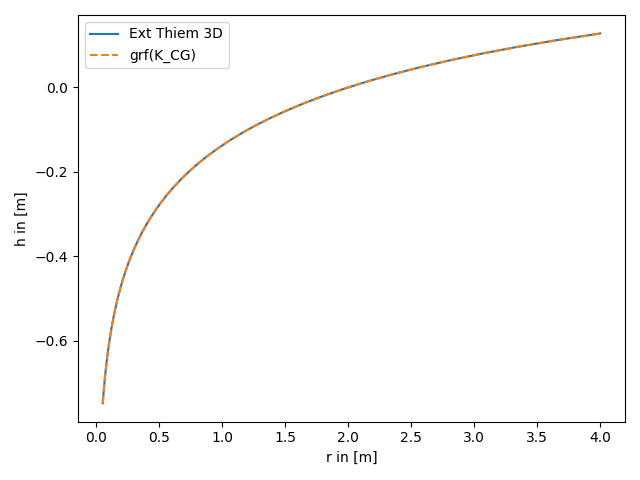# extended Thiem 3D vs. steady solution for coarse graining conductivity

The extended Thiem 3D solutions is the analytical solution of the groundwater flow equation for the coarse graining conductivity for pumping tests. Therefore the results should coincide.

Reference: Zech et. al. 2012```import numpy as np
from matplotlib import pyplot as plt

from anaflow.tools.coarse_graining import K_CG

rad = np.geomspace(0.05, 4)  # radius from the pumping well in [0, 4]
r_ref = 2.0  # reference radius

var = 0.5  # variance of the log-transmissivity
len_scale = 10.0  # correlation length of the log-transmissivity
KG = 1e-4  # the geometric mean of the transmissivity
anis = 0.7  # aniso ratio

rate = -1e-4  # pumping rate

r_ref,
K_CG,
rate=rate,
cond_gmean=KG,
var=var,
len_scale=len_scale,
anis=anis,
)